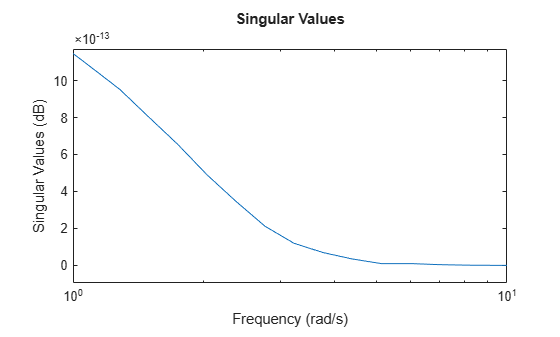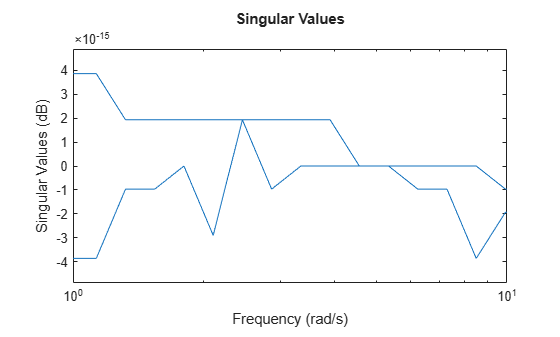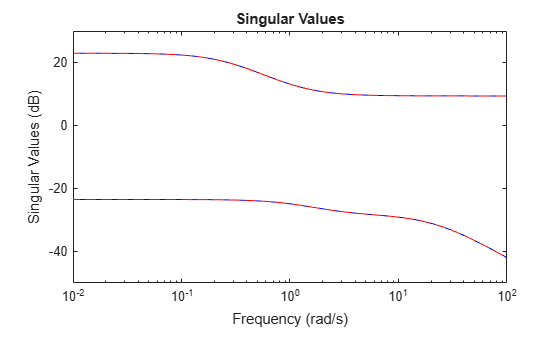# lncf

Left normalized coprime factorization

Since R2019a

## Syntax

``fact = lncf(sys)``
``[fact,Ml,Nl] = lncf(sys)``

## Description

example

````fact = lncf(sys)` computes the left normalized coprime factorization of the dynamic system model `sys`. The factorization is given by:$sys={M}_{l}^{-1}{N}_{l},\text{ }{M}_{l}{M}_{l}^{*}+{N}_{l}{N}_{l}^{*}=I.$Here, ${M}_{l}^{*}$ denotes the conjugate of Ml (see `ctranspose`). . The returned model `fact` is a minimal state-space realization of the stable system [Ml,Nl]. This factorization is used in other normalized coprime factor computations such as model reduction (`reducespec`) and controller synthesis (`ncfsyn`).```

example

````[fact,Ml,Nl] = lncf(sys)` also returns the coprime factors Ml and Nl.```

## Examples

collapse all

Compute the left normalized coprime factorization of a SISO system.

```sys = zpk([1 -1+2i -1-2i],[-1 2+1i 2-1i],1); [fact,Ml,Nl] = lncf(sys);```

Examine the original system and its factors.

`sys`
```sys = (s-1) (s^2 + 2s + 5) -------------------- (s+1) (s^2 - 4s + 5) Continuous-time zero/pole/gain model. ```
`zpk(Ml)`
```ans = 0.70711 (s+1) (s^2 - 4s + 5) ---------------------------- (s+1) (s^2 + 3.162s + 5) Continuous-time zero/pole/gain model. ```
`zpk(Nl)`
```ans = 0.70711 (s-1) (s^2 + 2s + 5) ---------------------------- (s+1) (s^2 + 3.162s + 5) Continuous-time zero/pole/gain model. ```

The numerators of the factors `Ml` and `Nl` are the denominator and numerator of `sys`, respectively. Thus, `sys = Ml\Nl`. `lncf` chooses the denominators of the factors such that the system $\left[{\mathit{M}}_{\mathit{l}}\left(\mathit{j}\omega \right),{\mathit{N}}_{\mathit{l}}\left(\mathit{j}\omega \right)\right]$ is a unit vector at all frequencies. To confirm that property of the factorization, examine the singular values of `fact`, which is a stable minimal realization of $\left[{\mathit{M}}_{\mathit{l}}\left(\mathit{j}\omega \right),{\mathit{N}}_{\mathit{l}}\left(\mathit{j}\omega \right)\right]$.

`sigma(fact)`Within a small numerical error, the singular value of `fact` is 1 (0 dB) at all frequencies.

Compute the left normalized coprime factorization of a state-space model that has two outputs, two inputs, and three states.

```rng(0); % for reproducibility sys = rss(3,2,2); [fact,Ml,Nl] = lncf(sys);```

`fact` is a stable minimal realization of the factorization given by `[Ml,Nl]`.

`isstable(fact)`
```ans = logical 1 ```

Another property of `fact` is that its frequency response F(jω) is an orthogonal matrix at all frequencies (F(jω)’F(jω) = I). Confirm this property by examining the singular values of `fact`. Within a small numerical error, the singular values are 1 (0 dB) at all frequencies.

`sigma(fact)`Confirm that the factors satisfy `sys = Ml\Nl` by examining the singular values of both.

`sigma(sys,'b-',Ml\Nl,'r--')`## Input Arguments

collapse all

Input system to factorize, specified as a dynamic system model such as a state-space (`ss`) model. If `sys` is a generalized state-space model with uncertain or tunable control design blocks, then the function uses the nominal or current value of those elements. `sys` cannot be an `frd` model or a model with time delays.

## Output Arguments

collapse all

Minimal realization of `[Ml,Nl]`, returned as a state-space model. `fact` is stable and its frequency response is an orthogonal matrix at all frequencies. If `sys` has `p` outputs and `m` inputs, then `fact` has `p` outputs and `m+p` inputs. `fact` has the same number of states as `sys`.

Left coprime factors of `sys`, returned as state-space models. If `sys` has `p` outputs and `m` inputs, then:

• `Ml` has `p` outputs and `p` inputs.

• `Nl` has `p` outputs and `m` inputs.

Both factors have the same number of states as `sys` and the same `A` and `C` matrices as `fact`.

## Tips

• `fact` is a minimal realization of `[Ml,Nl]`. If you need to use `[Ml,Nl]` or `[Ml,Nl]'` in a computation, it is better to use `fact` than to concatenate the factors yourself. Such manual concatenation results in extra (nonminimal) states, which can lead to decreased numerical accuracy.

## Version History

Introduced in R2019a